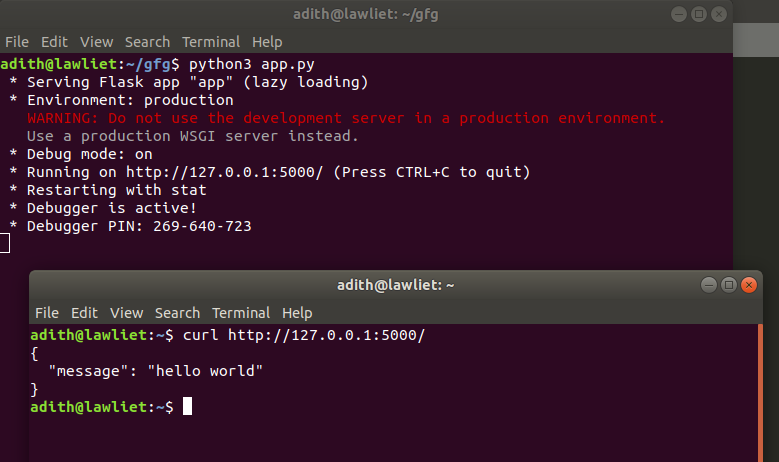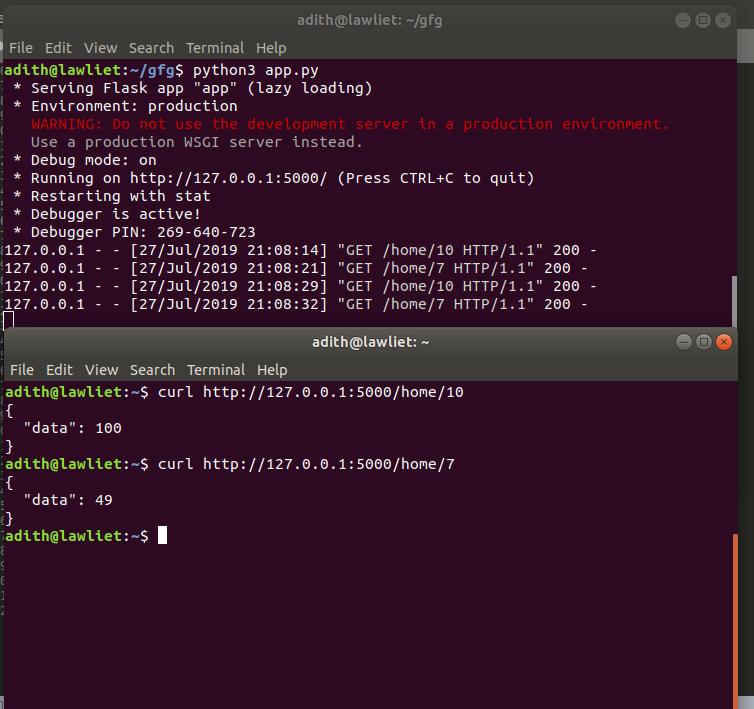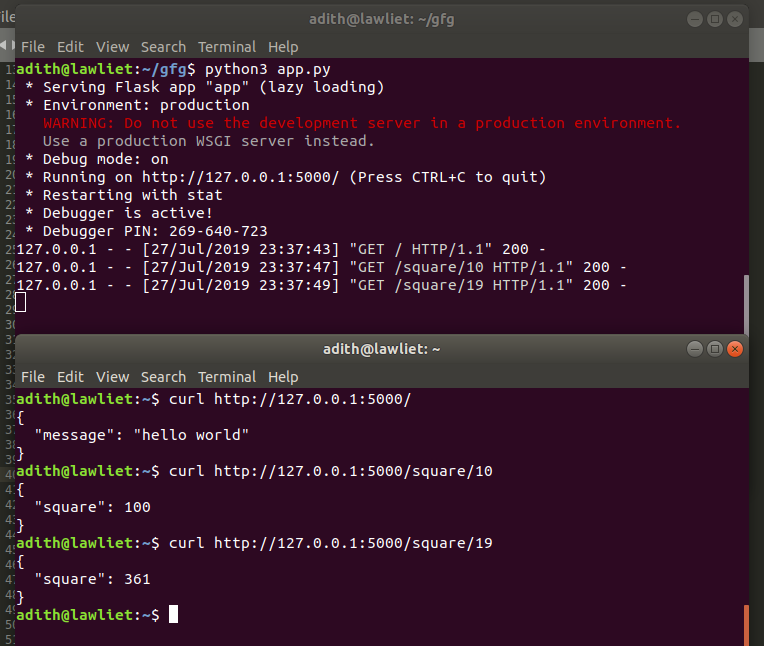# Python | Build a REST API using Flask

Prerequisite: Introduction to Rest API

REST stands for REpresentational State Transfer and is an architectural style used in modern web development. It defines a set or rules/constraints for a web application to send and receive data.

In this article, we will build a REST API in Python using the Flask framework. Flask is a popular micro framework for building web applications. Since it is a micro-framework, it is very easy to use and lacks most of the advanced functionality which is found in a full-fledged framework. Therefore, building a REST API in Flask is very simple.

There are two ways of creating a REST API in Flask:

1. Using Flask without any external libraries

## Libraries required:

`flask_restful `can be installed via the pip command:

`` sudo pip3 install flask-restful ``

## Method 1: using only Flask

Here, there are two functions: One function to just return or print the data sent through GET or POST and another function to calculate the square of a number sent through GET request and print it.

 `# Using flask to make an api ` `# import necessary libraries and functions ` `from` `flask ``import` `Flask, jsonify, request ` ` `  `# creating a Flask app ` `app ``=` `Flask(__name__) ` ` `  `# on the terminal type: curl http://127.0.0.1:5000/ ` `# returns hello world when we use GET. ` `# returns the data that we send when we use POST. ` `@app``.route(``'/'``, methods ``=` `[``'GET'``, ``'POST'``]) ` `def` `home(): ` `    ``if``(request.method ``=``=` `'GET'``): ` ` `  `        ``data ``=` `"hello world"` `        ``return` `jsonify({``'data'``: data}) ` ` `  ` `  `# A simple function to calculate the square of a number ` `# the number to be squared is sent in the URL when we use GET ` `# on the terminal type: curl http://127.0.0.1:5000 / home / 10 ` `# this returns 100 (square of 10) ` `@app``.route(``'/home/'``, methods ``=` `[``'GET'``]) ` `def` `disp(num): ` ` `  `    ``return` `jsonify({``'data'``: num``*``*``2``}) ` ` `  ` `  `# driver function ` `if` `__name__ ``=``=` `'__main__'``: ` ` `  `    ``app.run(debug ``=` `True``) `

Output:Executing the square function:Flask Restful is an extension for Flask that adds support for building REST APIs in Python using Flask as the back-end. It encourages best practices and is very easy to set up. Flask restful is very easy to pick up if you’re already familiar with flask.

In `flask_restful`, the main building block is a resource. Each resource can have several methods associated with it such as GET, POST, PUT, DELETE, etc. for example, there could be a resource that calculates the square of a number whenever a get request is sent to it. Each resource is a class that inherits from the Resource class of flask_restful. Once the resource is created and defined, we can add our custom resource to the api and specify a URL path for that corresponding resource.

 `# using flask_restful ` `from` `flask ``import` `Flask, jsonify, request ` `from` `flask_restful ``import` `Resource, Api ` ` `  `# creating the flask app ` `app ``=` `Flask(__name__) ` `# creating an API object ` `api ``=` `Api(app) ` ` `  `# making a class for a particular resource ` `# the get, post methods correspond to get and post requests ` `# they are automatically mapped by flask_restful. ` `# other methods include put, delete, etc. ` `class` `Hello(Resource): ` ` `  `    ``# corresponds to the GET request. ` `    ``# this function is called whenever there ` `    ``# is a GET request for this resource ` `    ``def` `get(``self``): ` ` `  `        ``return` `jsonify({``'message'``: ``'hello world'``}) ` ` `  `    ``# Corresponds to POST request ` `    ``def` `post(``self``): ` `         `  `        ``data ``=` `request.get_json()     ``# status code ` `        ``return` `jsonify({``'data'``: data}), ``201` ` `  ` `  `# another resource to calculate the square of a number ` `class` `Square(Resource): ` ` `  `    ``def` `get(``self``, num): ` ` `  `        ``return` `jsonify({``'square'``: num``*``*``2``}) ` ` `  ` `  `# adding the defined resources along with their corresponding urls ` `api.add_resource(Hello, ``'/'``) ` `api.add_resource(square, ``'/square/'``) ` ` `  ` `  `# driver function ` `if` `__name__ ``=``=` `'__main__'``: ` ` `  `    ``app.run(debug ``=` `True``) `

Output:My Personal Notes arrow_drop_upCheck out this Author's contributed articles.

If you like GeeksforGeeks and would like to contribute, you can also write an article using contribute.geeksforgeeks.org or mail your article to contribute@geeksforgeeks.org. See your article appearing on the GeeksforGeeks main page and help other Geeks.

Please Improve this article if you find anything incorrect by clicking on the "Improve Article" button below.

Article Tags :

Be the First to upvote.

Please write to us at contribute@geeksforgeeks.org to report any issue with the above content.